Function Repository Resource:

# FivePointConic

Find a conic equation that passes through five given points

Contributed by: Ed Pegg Jr
 ResourceFunction["FivePointConic"][pts,{x,y}] returns the implicit Cartesian equation in the variables x and y of the conic section that goes through the points pts. ResourceFunction["FivePointConic"][pts] uses the formal variables x and y.

## Details

For random, uniformly-distributed points in a rectangle, the probability of a hyperbola is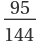and the probability of an ellipse is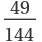.

## Examples

### Basic Examples (2)

Find a conic section through five points:

 In:=Out=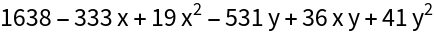Show the conic and points:

 In:=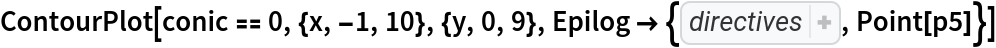Out=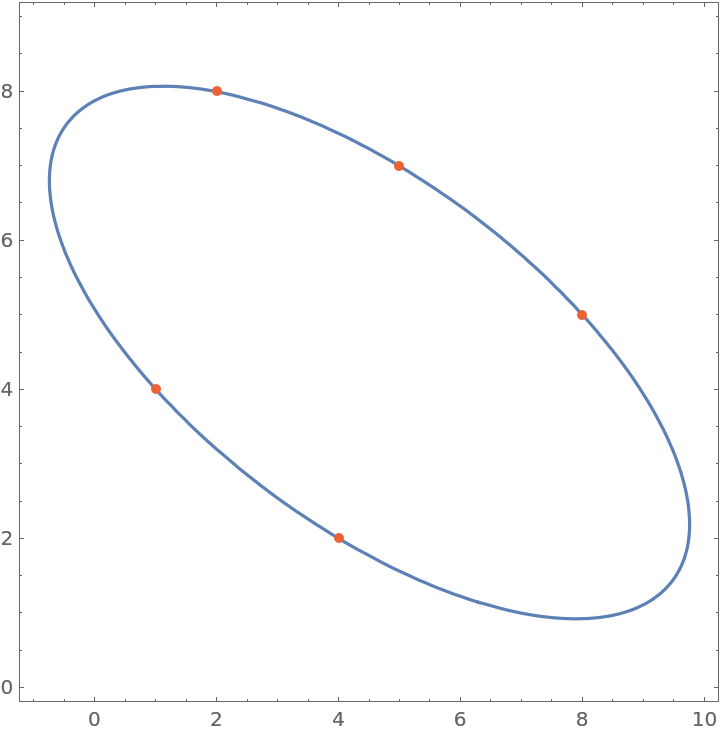### Scope (7)

Use formal variables:

 In:=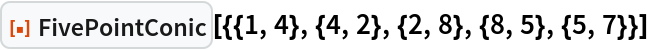Out=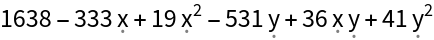The results of a five point conic are usually a hyperbola:

 In:=Out=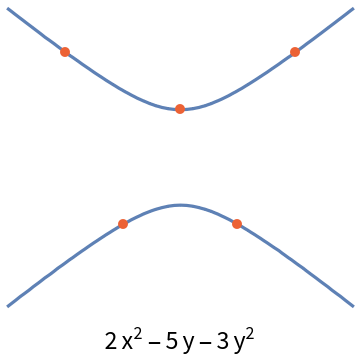A random five point conic is also frequently an ellipse:

 In:=Out=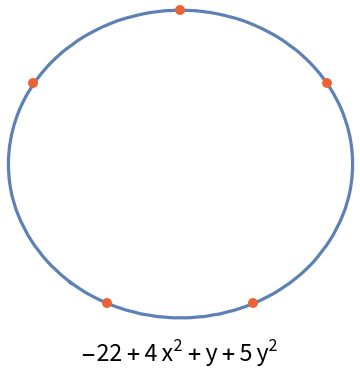A circle can also be the result of a five point conic:

 In:=Out=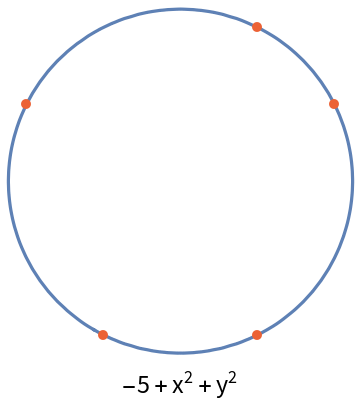A parabola may also appear:

 In:=Out=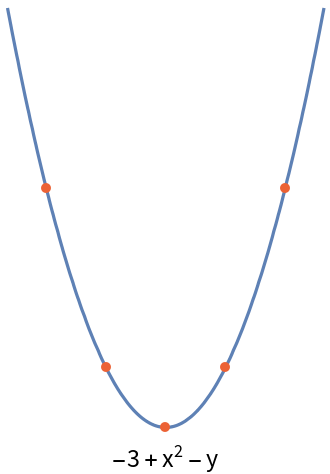Degenerate conics are also possible:

 In:=Out=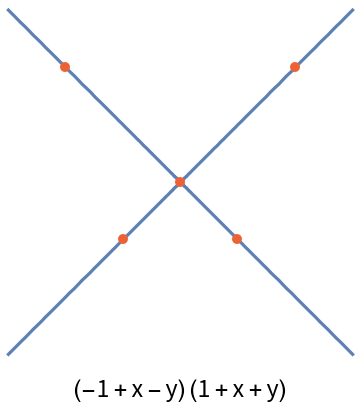See the properties of the non-degenerate conics with the resource function ConicProperties:

 In:=Out=### Neat Examples (5)

A function for characterizing a conic section:

 In:=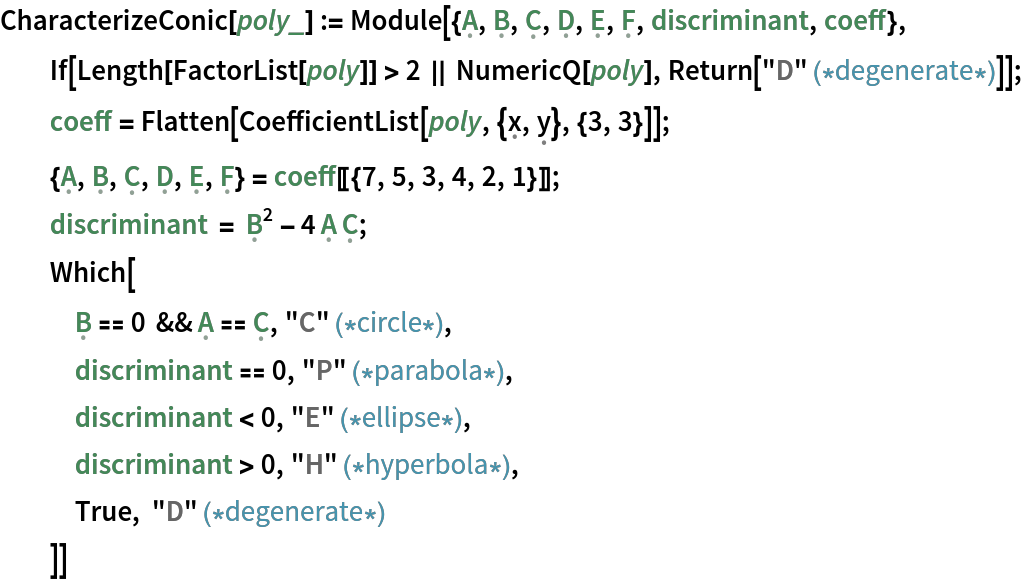A sample conic section:

 In:=Out=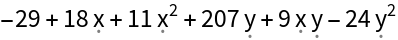The above polynomial defines a hyperbola:

 In:=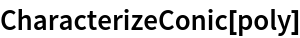Out=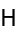Degenerate conics appear frequently when selecting from a limited lattice:

 In:=Out=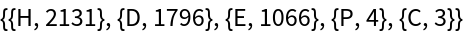For random real-valued points, the result is always a hyperbola or ellipse:

 In:=Out=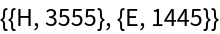## Version History

• 1.0.0 – 22 July 2022